• select 学号,姓名,课程名称,考试成绩,avg(考试成绩)平均成绩,max(考试成绩)最高成绩,min(考试成绩)最低成绩 from G1 group by 学号,姓名,课程名称,考试成绩; 结果如下 [img=...
• 查出每个学生对应 平均值再与原表连接，然后查询条件就比较该课程成绩分数和平均值 select t1.student_id,course_id from t_mark t1, ( SELECT student_id,AVG(mark) avg from t_mark group by student_id ...
情景
有一张表，里面是学生及其对应课程的成绩，要查出大于学生的所有课程平均值的课程。
表的结构解决方法
我想到两种sql，如下
第一种
查出每个学生对应的 平均值再与原表连接，然后查询条件就比较该课程成绩分数和平均值
select t1.student_id,course_id
from t_mark t1,
(
SELECT student_id,AVG(mark) avg
from
t_mark
group by student_id
) t2
where t1.student_id = t2.student_id
and t1.mark > t2.avg

第二种
每次在查询条件使用子查询查出该学生的课程平均值比较
select t1.student_id,course_id
from t_mark t1
where t1.mark>
(select AVG(mark)
from t_mark t2
where t2. student_id = t1.student_id)

比较两种sql
填充数据
为了有足够数据进行比较，写一个存储过程给3000个学生插入3条成绩数据
CREATE DEFINER=root@localhost PROCEDURE insert_to_mark()
BEGIN
#Routine body goes here...
DECLARE i INTEGER;
SET i = 1;
WHILE i<=3000 DO
INSERT INTO t_mark
(student_id,course_id,mark)
VALUES
(i,1,RAND()*70+30),
(i,2,RAND()*70+30),
(i,3,RAND()*70+30);
set i = i+1;
END WHILE;
END

插入成功查询比较
第一种第二种差距相当大。通过执行计划，看到第二个子查询是一个相关子查询（DEPENDENT SUBQUERY），第二个sql子查询中的参数是需要依赖外部查询，因此会对每个student_id 执行一次子查询，数据大时效率很低。
结论
还是使用第一种比较好，不知是否有更高效的查询sql欢迎评论 ~~


展开全文数据库 mysql sql
•  如何用SQL查询和李晓明同学平均成绩在同一分数段其他所有学生名单? 同一分数段为10分一分数段</p>sql 有问必答
• 1、查询你自己各门课的成绩：V_自己的成绩（学号、姓名、课程名、分数） ...2、查询你自己各门课的成绩：V_自己的成绩（学号、姓名、课程名、分数，要求这几字段用英文命名:Num,Name,CourseName,Score） create v
1、查询你自己各门课的成绩：V_自己的成绩（学号、姓名、课程名、分数）
create view v_自己的成绩
as
select sc.学号,姓名,课程名,分数 from  学生表 s , 课程表 c , 成绩表 sc
where s.学号=sc.学号 and c.ID=sc.课程ID and sc.学号='151011029'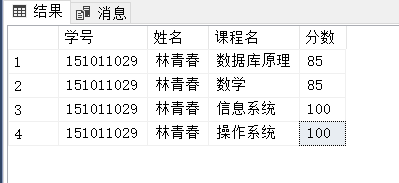2、查询你自己各门课的成绩：V_自己的成绩（学号、姓名、课程名、分数，要求这几个字段用英文命名:Num,Name,CourseName,Score）
create view v_自己的成绩(Num , Name , CourseName , Score)
as
select sc.学号,姓名,课程名,分数 from  学生表 s , 课程表 c , 成绩表 sc
where s.学号=sc.学号 and c.ID=sc.课程ID and sc.学号='151011029'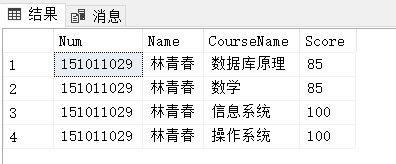3、统计每个同学的平均成绩：V_每人平均分（学号、姓名、平均分）,按平均分降序排列
create view v_每人平均分
as
select s.学号 , c.姓名 , avg(分数) as 平均分
from 成绩表 s,学生表 c
where s.学号=c.学号
group by s.学号,c.姓名
order by 平均分 desc

这里有问题，视图不能用order by 。会出现
“除非另外还指定了 TOP 或 FOR XML，否则，ORDER BY 子句在视图、内联函数、派生表、子查询和公用表表达式中无效。”
网上说添加 top 100 percent
但是我添加了之后可以执行，但是执行后并没有排序。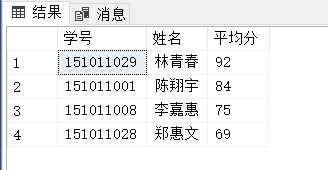4、建立统计每个系的学生人数视图：V_各系学生人数（系名、学生人数），按人数升序排列
create view v_各系学生人数(系名,学生人数)
as
select top 100 percent s.系部ID , count(*)as 学生人数
from 学生表 s ,系部表 c
where s.系部ID = c.系部ID
group by s.系部ID
order by 学生人数 asc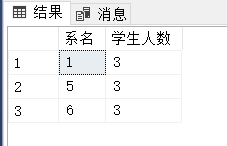5、建立计算机系学生的视图：V_计算机系学生。要求能看到学生的学号、姓名、性别和联系电话。
create view v_计算机系学生
as
select 学号 ,姓名 ,性别, 联系电话
from 学生表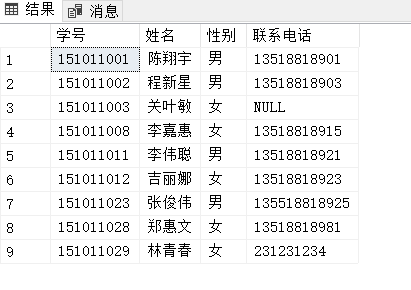6、删除统计每个系的学生人数R 视图：V_各系学生人数
drop view v_各系学生人数



展开全文• 给出n个学生的m门课程的成绩表，每个学生的信息由学号、姓名以及各科成绩组成。对学生的考试成绩进行有关统计分析，并打印统计表。 基本要求： 1、 通过键盘输入各学生的多门课程的成绩，建立相应的文件input.dat。 ...
成绩统计分析系统
文章目录成绩统计分析系统源代码github地址一、要求二、系统功介绍三、数据结构设计四、系统实现（关键代码）1、系统首页2、系统录入学生3、系统选择各项操作4、系统排序操作5、系统查询—学号6、系统查询—姓名操作7、系统成绩分析8、学生添加
源代码github地址
Score_System.git
一、要求
问题描述：
给出n个学生的m门课程的成绩表，每个学生的信息由学号、姓名以及各科成绩组成。对学生的考试成绩进行有关统计分析，并打印统计表。
基本要求：
1、	通过键盘输入各学生的多门课程的成绩，建立相应的文件input.dat。
2、	对文件input.dat中的数据进行处理，要求具有如下功能：
i.	按各门课程成绩排序，并生成相应的文件输出。
ii.	计算每人的平均成绩，按平均成绩排序，并生成文件。
iii.	求出各门课程的平均成绩、最高分、最低分、不及格人数、6069分人数、7079分人数、80~89分人数、90分以上人数。
iv.	根据姓名或学号查询某人的各门课成绩，重名情况也能处理。
3、	界面美观。
测试数据
学号	姓名	数学	英语	计算机

001
王放
78
77
90

002
张强
89
67
88

003
李浩
56
66
78

004
黄鹂兵
89
86
85

005
李浩
67
88
76

006
陈利风
45
54
67

007
尚晓
78
76
70

4 选做内容  对各科成绩设置不同的权值。
二、系统功介绍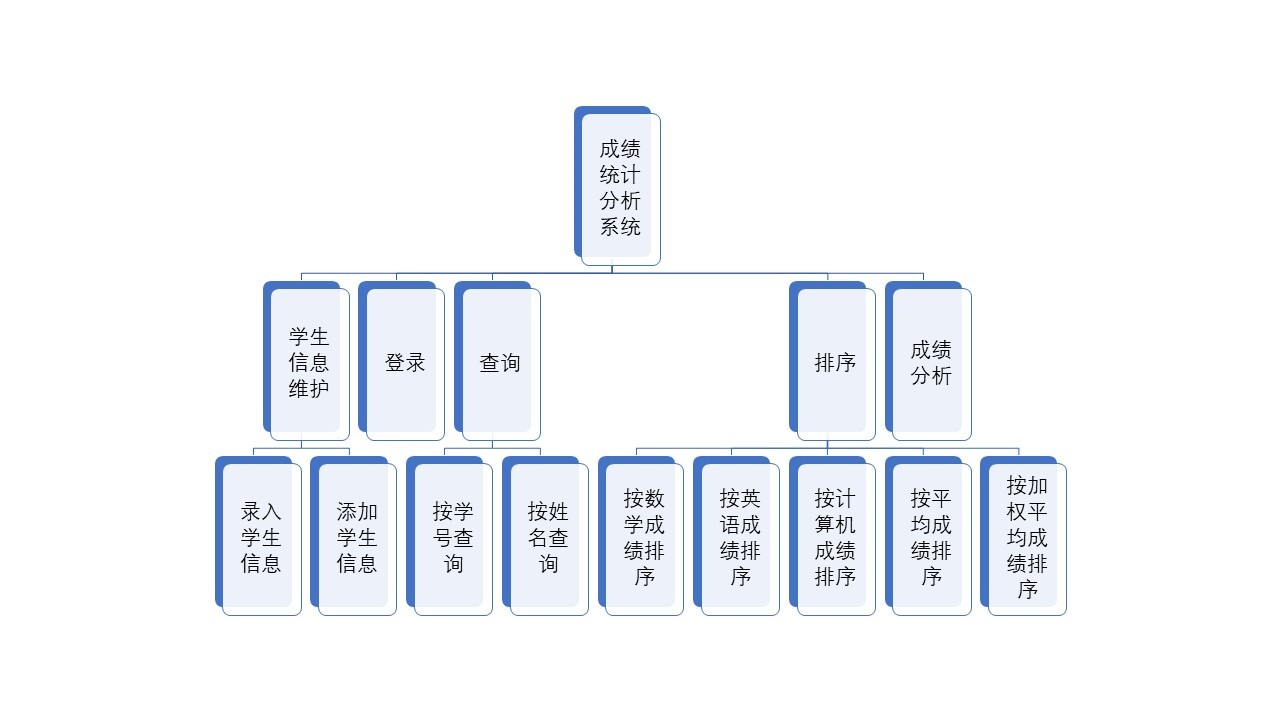系统功能包括学生信息维护，登录，查询，排序，成绩分析具体说明如下：

学生信息维护：主要完成进入系统后的学生信息录入及在操作过程中需要的学生信息添加。
登录：用户登录，只有登录成功的用户才能使用本系统功能
查询：管理员能够按照不同信息（学号、姓名）进行查询。
排序：管理员能够按照不同需求（各科成绩、平均成绩、加权平均成绩）进行查询
成绩分析：输出各门课程的平均成绩、最高分、最低分、不及格人数、6069分人数、7079分人数、80~89分人数、90分以上人数。

三、数据结构设计
学生对象：
struct STUDENT
{
int studentNo; //学号
char name; //姓名
float scores;//各科成绩
float ravg_score; //加权后的平均成绩
float avg_score; //不加权的平均成绩
};


四、系统实现（关键代码）
1、系统首页
用户未登录时可进入的首页，在首页可以进行用户的登录
while (1)
{
printf("\n\n                    ^^^^^^^^^^^^^^^^^^^^^^^^^^^\n");
printf("                    $请输入管理员账号：$\n");
printf("                     ");
scanf("%d", &account);
printf("                    $请输入密码：$\n");
printf("                     ");
scanf("%d", &pass);
printf("\n                    ^^^^^^^^^^^^^^^^^^^^^^^^^^^\n\n");
if (account == 111 && pass == 123)
{
break;
}
else
{
printf("                    输入信息有误请重新输入！\n");
}
}

2、系统录入学生
输入学生人数及调用函数部分：
	STUDENT stu[MAX];
float rat;
printf("\n\n                     ^^^^^^^^^^^^^^^^^^^^^^^^^^^^^^^^^^^^^^^^^^^^^^^\n");
printf("                    $请输入学生人数(小于100)$\n                    ");
scanf("%d", &n);
printf("\n                    ^^^^^^^^^^^^^^^^^^^^^^^^^^^^^^^^^^^^^^^^^^^^^^^\n\n");
input(stu, n, m);
input_ratios(rat);
getAvgScore(stu, rat, n, m);
write(stu, n, "input.data");


输入每个学生信息的关键代码为：
for (i = 0; i < n; i++)
{
printf("                    输入第%d个学生的信息：\n",i+1);
printf("                    学号：\n                    ");
scanf("%d", &students[i].studentNo);
printf("                    姓名：\n                    ");
scanf("%s", &students[i].name);
for ( j = 0; j < m; j++)
{
printf("                    第%d科成绩：\n                    ", j + 1);
scanf("%f", &students[i].scores[j]);
}
printf("                    ——————————————————————————————\n");
}


输入各科权重的关键代码为：
	fp = fopen("ratios.data", "wb");
if (fp == NULL)
{
printf("Can't open the file");
}
printf("\n\n                    ^^^^^^^^^^^^^^^^^^^^^^^^^^^^^^^^^^^^^^^^^\n\n");
printf("                    请输入各科权重（和为1且大于0小于1）：\n\n");
while (1)
{
printf("                    数学成绩的权重为：\n                    ");
scanf("%f", &rat);
if (rat >= 0 && rat <= 1)
{
break;
}
else
{
printf("                    数学成绩权重输入有误！\n\n");
}
}
while (1)
{
printf("\n                    英语成绩的权重为：\n                    ");
scanf("%f", &rat);
if (rat >= 0 && rat <= 1 && (rat + rat) <= 1)
{
break;
}
else
{
printf("                    英语成绩权重输入有误！\n\n");
}
}
rat = 1.0 - rat - rat;
for (i = 0; i < 3; i++)
{
fwrite(&rat[i], sizeof(float), 1, fp);
//写入权重
}
fclose(fp);


3、系统选择各项操作
用户根据自己的需求选择相应的操作，后台通过验证其输入操作是否有误，返回相关信息。
	printf("\n\n                    请输你的选择：");
scanf("%d", &opse);
while (opse < 0 || opse > 9)
{
printf("\n                    输入有误，请重新输你的选择：\n                    ");
scanf("%d", &opse);
}

4、系统排序操作
用户选择排序的标准（1-学号、2-数学成绩、3-英语成绩、4-计算机成绩、5-平均成绩、6-加权平均成绩）系统自动输出降序排序的结果。
	int left = startIndex;
//把第一个元素作为基准元素
STUDENT &pivot = stu[startIndex];
int right = endIndex;
while (left != right)
{
/*比较各科目成绩*/
if (k >= 1 && k <= 3)
{
//控制right右移动
while (left < right && stu[right].scores[k - 1] <= pivot.scores[k - 1])
{
right--;
}
//left左移
while (left < right && stu[left].scores[k - 1] >= pivot.scores[k - 1])
{
left++;
}
}
/*比较平均成绩*/
if (k == 4)
{
//控制right右移动
while (left < right && stu[right].avg_score <= pivot.avg_score)
{
right--;
}
//left左移
while (left < right && stu[left].avg_score >= pivot.avg_score)
{
left++;
}
}

/*比较加权平均成绩*/
if (k == 5)
{
//控制right右移动
while (left < right && stu[right].ravg_score <= pivot.ravg_score)
{
right--;
}
//left左移
while (left < right && stu[left].ravg_score <= pivot.ravg_score)
{
left++;
}
}

/*比较学号*/
if (k == 6)
{
//控制right右移动
while (left < right && stu[right].studentNo > pivot.studentNo)
{
right--;
}
//left左移
while (left < right && stu[left].studentNo > pivot.studentNo)
{
left++;
}
}

//交换left和right所在位置的值
if (left < right)
{
exchange(&stu[left], &stu[right]);
}
}
//当left=right 交换基准元素和该位置（left或者right）的值
exchange(&stu[left], &pivot);
return left;


5、系统查询—学号
二分法：
	int low, high, mid;
STUDENT stu_null;
stu_null.studentNo = -1;
low = 0;
high = length - 1;
while (low <= high)
{
mid = (low + high) / 2;
if (stu[mid].studentNo == key)
{
return stu[mid];
}
if (stu[mid].studentNo > key)
{
high = mid - 1;
}
if (stu[mid].studentNo < key)
{
low = mid + 1;
}
}
return stu_null;

6、系统查询—姓名操作
用户可以选择根据姓名查询对应学生，（重名情况可处理）
	for ( i = 0; i < n; i++)
{
if (strcmp(name,stu[i].name) == 0)
{
flag = 1;
count++;
printf("                    第 %d 个学生:\n\n                    学号：%d\t姓名：%s\t数学成绩：%.1f\t英语成绩：%.1f\t计算机成绩：%.1f\t平均分：%.1f\t加权平均分%.1f\n",
count,stu[i].studentNo, stu[i].name, stu[i].scores,
stu[i].scores, stu[i].scores, stu[i].avg_score, stu[i].ravg_score);
}
}
if (flag == 0)
{
printf("                    查找的学生不存在！");
}


7、系统成绩分析
用户选择成绩分析选项，系统可根据输入学生进行各科成绩分析
	for (i = 0; i < n; i++)
{
//每科总成绩
math_result += students[i].scores;
english_result += students[i].scores;
cs_result += students[i].scores;

//分数段统计
//数学
switch (degree(students[i].scores))
{
case 0:
math_result++;
break;
case 1:
math_result++;
break;
case 2:
math_result++;
break;
case 3:
math_result++;
break;
case 4:
math_result++;
}

//英语
switch (degree(students[i].scores))
{
case 1:
english_result++;
break;
case 2:
english_result++;
break;
case 3:
english_result++;
break;
case 4:
english_result++;
break;
}
//计算机
switch (degree(students[i].scores))
{
case 0:
cs_result++;
break;
case 1:
cs_result++;
break;
case 2:
cs_result++;
break;
case 3:
cs_result++;
break;
case 4:
cs_result++;
break;
}
}
//各门课统计最高分、最低分

//数学
Quick_Sort(students, 0, n, 1);
stu_max = students;
stu_min = students[n - 1];
math_result = stu_max.scores;
math_result = stu_min.scores;

//英语
Quick_Sort(students, 0, n, 2);
stu_max = students;
stu_min = students[n - 1];
english_result = stu_max.scores;
english_result = stu_min.scores;

//计算机
Quick_Sort(students, 0, n, 3);
stu_max = students;
stu_min = students[n - 1];
cs_result = stu_max.scores;
cs_result = stu_min.scores;

//平均分
math_result = math_result / n;
english_result = english_result / n;
cs_result = cs_result / n;


8、学生添加
在程序运行过程中，用户可以根据需求添加学生，需要输入添加人数及添加各个学生的信息
fp = fopen("ratios.data", "rb");
if (fp == NULL)
{
printf("Can't open the file");
}
for (i = 0; i < 3; i++)
{
fread(&ratios[i], sizeof(float), 1, fp);
}
printf("\n\n                    ^^^^^^^^^^^^^^^^^^^^^^^^^^^^^^^^^^^^^^^^^^^^^^^\n");
printf("                    $请输入新增学生人数(小于100)：$\n                    ");
scanf("%d", &m);
printf("\n                    ^^^^^^^^^^^^^^^^^^^^^^^^^^^^^^^^^^^^^^^^^^^^^^^\n\n");
input(stu,m,3);
getAvgScore(stu, ratios, m, 3);
add(stu, m, "input.data");
n = m + n;




展开全文数据结构
• ②计算每个的成绩，总分数，平均分，要求显示学号，姓名，语文，数学，英语，总分，平均分;③列出数学成绩的排名（要求显示字段：学号，姓名，成绩，排名）。资源里展现了从创建数据库到查询的全部过程。
• 2.查询与“刘一平”来自同一的学生姓名 Select sname from student where sdept in ( select sdept from student where sname=‘刘晨’) and sname <> ‘刘晨’; 3.查询Sc表中最高分的学生..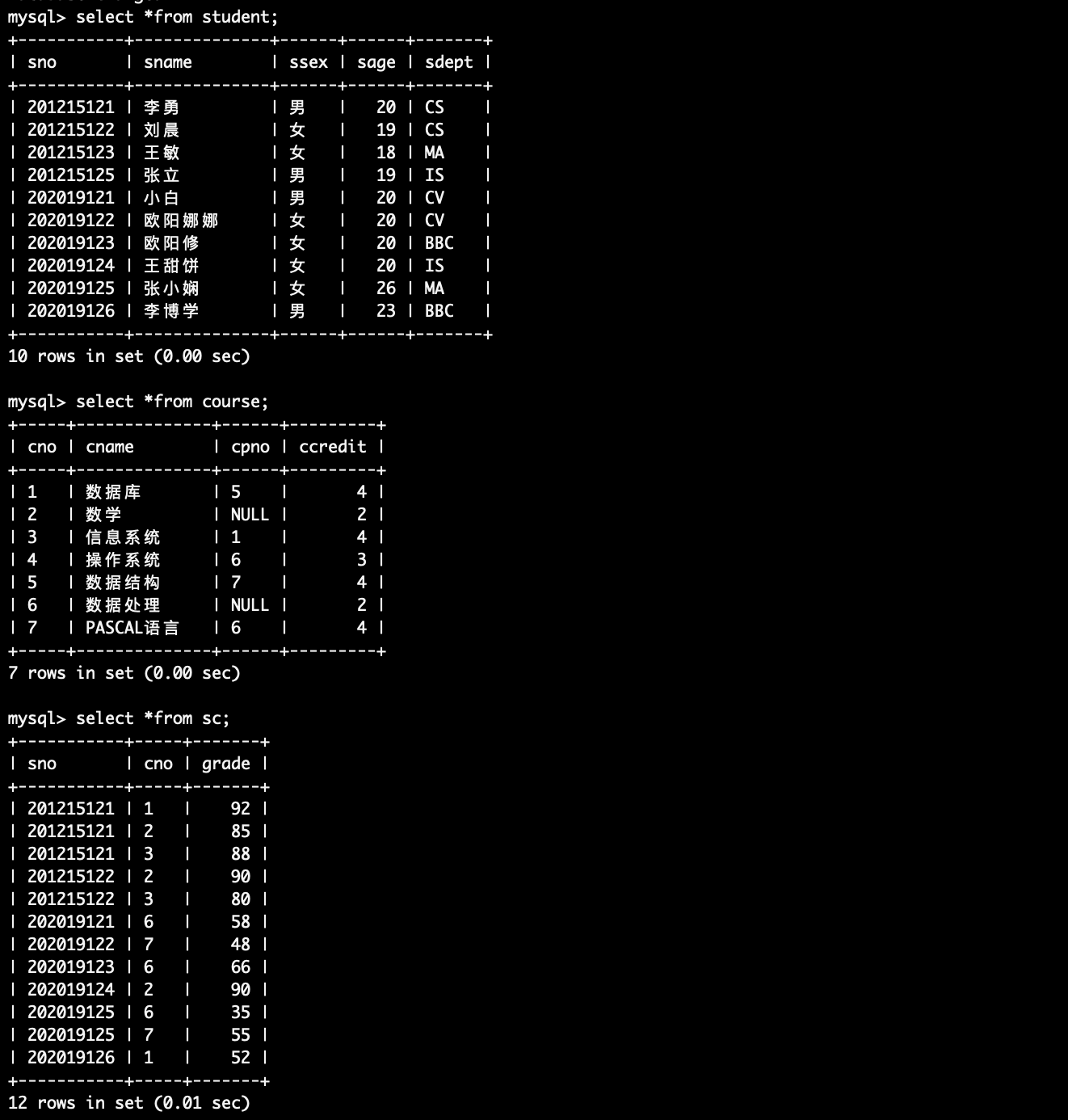1.查询选修了课程号为“3”的学生姓名和系别
Select sname,sdept from student where sno in (
select sno from sc where cno=‘3’);
2.查询与“刘一平”来自同一个系的学生姓名
Select sname from student where sdept in (
select sdept from student where sname=‘刘晨’) and sname <> ‘刘晨’;
3.查询Sc表中的最高分的学生学号和课程号
select sno,cno from sc where grade in(
select max(grade) from sc);
另一种：
select sno,cno from sc where grade >=all(
select grade from sc);
4.查询其它系中‘2‘号课程比信息系所有学生分数高的学生学号和姓名；
select distinct student.sno,sname from student,sc where student.sno=sc.sno and cno=‘2’ and grade >all (
select grade from sc where sdept=‘is’ ) and sdept <> ‘is’;
改进：
select distinct student.sno,sname from student,sc where student.sno=sc.sno and cno=‘2’ and grade >all ( select grade from sc where sno in(select sno from student where sdept = ‘is’) ) and sdept <> ‘is’;
老师：
select sno,sname from student where sno in (select sno from sc where cno=‘2’ and sno not in(select sno from student where sdept=‘cs’) and grade>(select max(grade) from sc where cno=‘2’ and sno in(select sno from student where sdept=‘cs’)));
5.查询其它系中比信息系所有学生年龄大的学生姓名和性别；
Select distinct sname,ssex from student where sage >all (
select sage from student where sdept = ‘is’ ) and sdept <> ‘is’;
6.查询每门课程中低于该课程平均成绩的学生学号和姓名；
Select student.sno,sname from student,sc x where student.sno = x.sno and grade < (
select avg(grade) from sc y where y.cno=x.cno ) ;
7.查询“信息系”中选课最多的学生学号；
select student.sno from student,sc where sc.sno=student.sno and student.sdept=‘is’ group by sc.sno having count() >= all(
select count() from sc, student where sc.sno= student.sno and sdept=‘is’ group by sc.sno);
select sno from student where sdept=‘cs’ and sno in(select sno from sc group  by sno having count()>=all(select count() from sc where sno in(select sno from student where sdept=‘cs’)group by sno));
8.查询所有选修“计算机导论”课程的“男”同学的成绩表
select student.sno,sname,course.cno,cname,grade from student,sc,course where student.sno=sc.sno and course.cno=sc.cno and ssex=‘男’ and student.sno in(select sno from sc,course where sc.cno=course.cno and cname = ‘计算机导论’);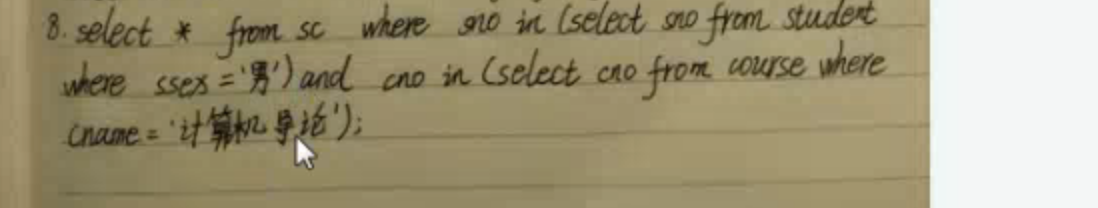9.查询有两门及以上课程不及格的学生学号和姓名；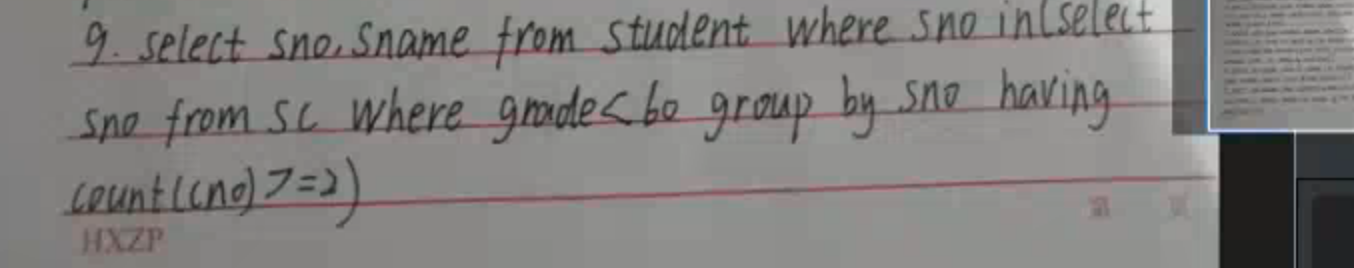10.查询比“2”号课程平均分高的其它课程信息；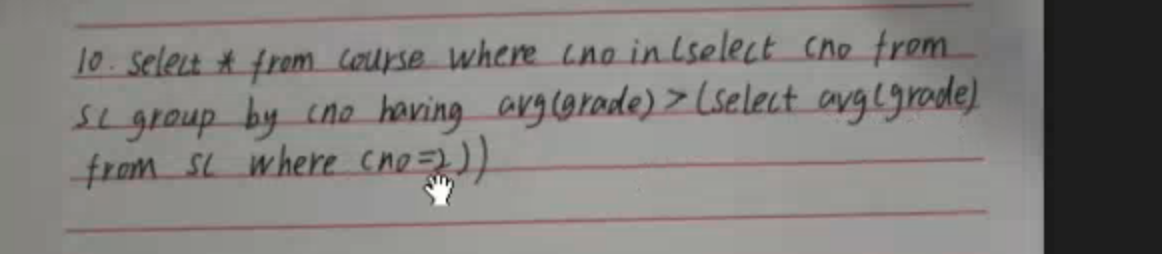展开全文• 对一个有N个学生的班级，每个学生有M门课程。该系统实现对班级成绩的录入、显示、修改、排序、保存等操作的管理。功能要求： （1）本系统采用一个结构体数组，每个数据的结构应当包括：学号、姓名、M门课程名称。 ...
• 设计一个Windows应用程序，在该程序中定义一个学生类和班级类，以处理每个学生的学号、姓名、语文、数学和英语3门课程的期末考试成绩，要求： （1）能根据姓名查询指定学生的总成绩。 （2）能统计全班学生的平均...C#
• ## 一个C#的学生成绩管理系统作业

万次阅读 多人点赞 2018-03-18 20:26:46
老师要求：能查询每个学生的总成绩、显示前三名成绩、单科成绩最高分和不及格的学生名单、全班平均成绩、各科成绩在不同分数段的学生人数百分比。第一次用C#，写的比较糙，但总的来说还是学到不少的：1.学会了...C#
• ** 学生成绩管理系统 **\n");  printf("** 作者：*** **\n");  printf("** **\n");  printf("** 增加学生信息 ---1 **\n");  printf("** 修改...有问必答 c语言
• 问题分析 设某班有10位同学，位同学有5门课成绩，要求程序包括4方面的...（2）根据学生的学号查询学生的总成绩和平均成绩 （3）根据学生学号和科目号查询某单科的成绩 （4）根据科目号统计单科成绩的总分和平均分
• （2）能统计全班学生的平均成绩。 （3）能统计单科成绩最高分。 （4）能统计全班前3名的名单 （5）能统计指定课程不及格的学生名单。 (6)能统计指定课程在不同分数段的学生人数百分比。 2.设计提示: (1)定义...c# 程序人生 经验分享
• C#程序设计 设计一Windows...（2）能统计全班学生的平均成绩 （3）能统计单科成绩的最高分 （4）能统计全班前三名的名单 （5）能统计指定课程不及格学生名单 （6）能统计课程在不同分数段的学生人数百分比 窗口界面1024程序员节
• (2) 计算每个学生的平均成绩和总成绩； (3) 按总成绩从高到低排名，并按名次输出每个学生的情况，包括： 学号，各科成绩，平均成绩，总成绩，排名 (4) 由键盘输入课程号，输出该课程成绩在90分（含90分）以上且...
• MATLAB实现学生成绩查询系统 部分源码 with handles and user data (see GUIDATA) yingyu=str2num(handles.chengjibiao.yingyu); %获得每个英语分数 [m,n]=size(yingyu); %获取人数，为m yy_pj=sum(yingyu)/m; %...
• 按提示输入个学生的信息，当生成相应对象时请给出相应的确认消息(构造函数实现)； 3.按行输出一个班学生的所有信息，及三门课的总分； 4.输出这个班的统计信息：如这个班的总人数，这三门课门课的平均成绩...
• 查询每个学生的平均成绩，输出平均成绩>85的学生的学号和平均成绩7.查询和林艳在同一个专业学习的女同学的学号与姓名。8.查询比数学专业所有学生年龄都小的其他专业的学生的学号、姓名9.查询选sql sqlserver
• 教师可以查看、修改、删除、录入学生成绩，可以统计每个学生的平均成绩和不及格的科目，还可以修改自己的密码。用户登录系统后再能进行成绩的查看、修改、删除、录入等操作，根据不同的角色，分配不同的权限。PHP
• 平均成绩的计算：计算每个学生各门功课的平均成绩，并按平均成绩从高到低的次序输出学生信息； 列出不及格学生清单（学号、姓名、不及格的课程和成绩）。 考虑用文件把数据保存起来（可选）。
• 功能：成绩管理系统包含了学生的全部信息，每个学生是一个记录，包括学号，姓名，性别，各科成绩等。系统可完成：信息录入——录入学生成绩信息（包括学生学号、姓名、各门课程的成绩等）；信息查询——输入学号，...
• （2）统计每个的平均成绩； （3）统计各科平均成绩。 二、用户操作方法 一共有七个源文件，一个头文件，六个cpp文件。 将它们打开编译后即可运行。 运行后按照提示操作即能实现预期的各种功能。 或者双击debug图标......